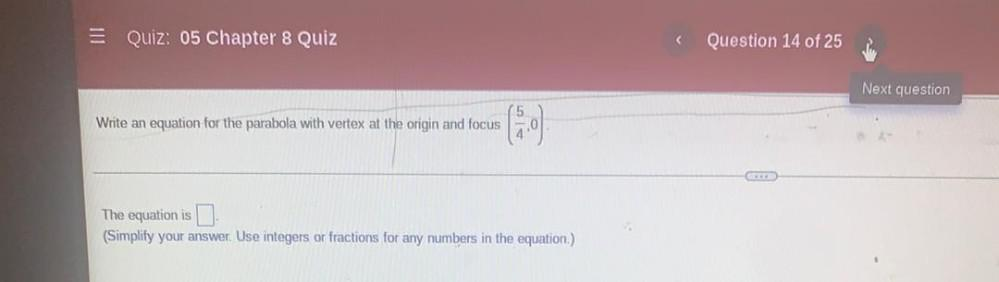Question:

# = Quiz: 05 Chapter 8 Quiz Question 14 of 25 Next question Write an equation for the parabola with vertex at the origin and focus= Quiz: 05 Chapter 8 Quiz Question 14 of 25 Next question Write an equation for the parabola with vertex at the origin and focus The equation is I (Simplify your answer. Use integers or fractions for any numbers in the equation.)